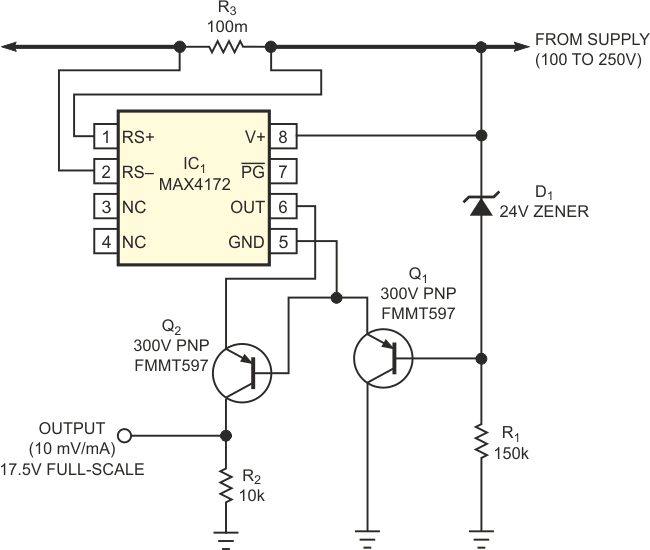# High-side current monitor operates at high voltage

## Maxim MAX4172

Greg Sutterlin, Brian Whitaker - Maxim Integrated Products

EDN

The simplest technique for measuring current in an actuator or a motor is to monitor the ground current with a resistive element between the load and the ground. Because the device and its associated electronics share a ground potential, you need to amplify only the ground-current signal. This approach, however, does not detect device short circuits to ground, which can overload the high-side drive circuitry. To avoid such potential fault conditions, you should use a high-side current monitor to detect short circuits and similar faults that can occur following the current monitor. High-side current monitoring has advantages, but it finds limited use because of the dearth of devices able to handle the high voltage levels – 24 V to many hundreds of volts – prevalent in the industry. Off-the-shelf devices can operate to 32 and 76 V, but even 76 V is insufficient for many applications. Figure 1 shows a simple way to adapt a standard 32 V device for use at any voltage level, subject to limitations of the external components. (The components in Figure 1 can accommodate 130 V.)Figure 1. This circuit enables a 36 V current-monitoring IC to operate at common-mode voltages as high as 130 V.

The accuracy of the circuit is better than 1% for load currents greater than 30 mA. IC1’s current-output stage allows easy implementation of the current mirror needed for level-shifting the output signal to ground. Thus, you can easily monitor the ground-referenced signal by using an A/D converter or a comparator. The circuit monitors load current in the presence of a 130 V-dc common-mode level. You must ensure that you do not violate IC1’s absolute maximum rating – 36 V with respect to the ground pin – for the RS+, RS–, and V+ pins. For that purpose, zener diode D1 limits the voltages between the V+, RS+, and GND pins to 24 V. Thus, the typical voltage between these pins is 24 V minus the VBE of Q1, or 23.3 V. The zener-diode current for this circuit is approximately 700 µA. Note that the manufacturer’s suggested bias current is 500 µA, but the zener diode’s di/dt slope goes negative below 300 µA, a condition that can introduce noise or even oscillation. The minimum specified bias – 300 to 500 µA – sets the maximum value of R1, and the maximum allowed power dissipation for R1 and D1 combined sets the minimum value for R1. Thus, for supply rails of 100 to 250 V, a reasonable R1 value is 150 to 225 kΩ – 150 kΩ in this case.

Q1 and R1 form a shunt regulator. The design uses Q1 because of its maximum VCE rating of –300 V, high gain of 100 V/V at 1 mA, and its ability to handle 500 mW of power. Output current is proportional to the voltage difference, VSENSE, between RS+ and RS–:whereTransconductance for IC1 is 10 mA/V. If the maximum monitored load current, ILOAD, is 4 A, and RSENSE is 10 mΩ, then the maximum output current isThus, IOUT is proportional to ILOAD, and the maximum expected output is 400 µA. For applications of wide dynamic range in which VSENSE can approach the absolute maximum rating of the differential pair, 700 mV, you should protect the sense pins by adding series resistors between RSENSE and RS+ and between RSENSE and RS–. You should select the resistor values to limit input currents to within 10 mA when the RS+ to RS– difference is 700 mV.

IOUT is now proportional to ILOAD, but, for easy monitoring, you must level-shift it to ground by using the Q1-Q2 current mirror. Q2’s high gain forces the collector current to closely approximate the emitter current which, when you apply it to R2, produces a measurable voltage at VOUT. As with Q1, Q2 needs a maximum VCE rating of –240 V. The device in Figure 1 is rated at –300 V. VOUT now equals IOUT × R2. (The actual output current at Q2’s collector is slightly less, because of Q2’s base current.) At ILOAD = 4 A,You can accommodate designs with lower or higher operating voltages by properly selecting Q1, Q2, and the base resistor, R1.

EDN